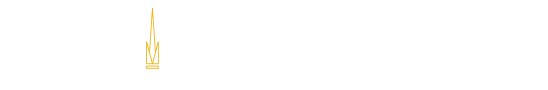### ECE Course Outline

#### Numerical Methods for Optimization and Optimal Control (3-0-3)

Prerequisites
ECE 3084 Signals and Systems OR ECE 3550 Feedback Control Systems
Corequisites
None
Catalog Description
Algorithms for numerical optimization and optimal control, Gradient-descent techniques, linear programming, numerical linear system solvers, second-order methods for optimizing performance of dynamical systems.
Textbook(s)
No Textbook Specified.
Course Objectives - As part of this course, students:

1. To teach students the elements of numerical and computational methods for optimization and optimal control.
2. Students will become familiar with static optimization methods such as gradient descent and Newton?s method, and with optimization of dynamical system
Course Outcomes - Upon successful completion of this course, students should be able to:

1. Students will be able to write computer code to implement linear system constructs learned in prior S+C courses.
2. Students will understand the types of errors than can arise in these implementations, and how to quantify them.
3. Students will be able to construct computer algorithms to solve other problems which don't benefit from closed form constructs acquired in previous
4. Students will know which types of models should be paired with which types of numerical optimization algorithms based on properties such as convexi
5. In cases were multiple choices of numerical optimization algorithms are suitable for a given problem, students will understand the relative advanta
Topical Outline
```I. Numerical Methods
I.1 Review of numerical issues when using machine number systems
I.2 Direct Linear systems solvers (LU, Cholesky, iterative refinement)
I.3 Scalar nonlinear equation solvers (bisection, Newton, secant method)
I.4 Numerical differentiation and Integration

II. Optimization
II.1. Introduction to nonlinear programming
II.2. Optimality conditions for constrained and unconstrained problems
II.4. Least-square problems and algorithms
II.5. Optimization problems with equality and inequality constraints

III. Algorithms for optimal control problems
III.1. Discrete-time optimal control: necessary optimality conditions
III.2. Continuous-time optimal control: the Hamiltonian
III.3. The linear-quadratic optimal control problem and feedback-based solution
III.4. The Pontryagin?s Maximum principle
III.5. Examples: minimum-time problems and minimal-path problems
```Georgia Institute of Technology
North Avenue, Atlanta, GA 30332
Phone: 404-894-2000